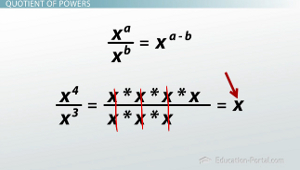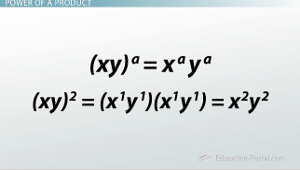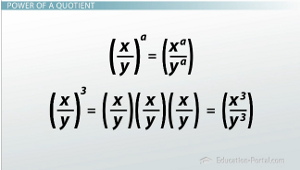# What Are the Five Main Exponent Properties?

An error occurred trying to load this video.

Try refreshing the page, or contact customer support.

Coming up next: How to Define a Zero and Negative Exponent

### You're on a roll. Keep up the good work!

Replay
Your next lesson will play in 10 seconds
• 0:30 Product of Powers
• 1:10 Power to a Power
• 1:50 Quotient of Powers
• 2:53 Power of a Product
• 3:40 Power of a Quotient
• 4:41 Lesson Summary

Want to watch this again later?

Timeline
Autoplay
Autoplay
Speed

#### Recommended Lessons and Courses for You

Lesson Transcript
Instructor: Kathryn Maloney

Kathryn teaches college math. She holds a master's degree in Learning and Technology.

We'll look at the five important exponent properties and an example of each. You can think of them as the order of operations for exponents. Learn how to handle math problems with exponents here!

## Understanding the Five Exponent Properties

We are going to talk about five exponent properties. You can think about them as the order of operations for exponents. Just like the order of operations, you need to memorize these operations to be successful. The five exponent properties are:• Product of Powers
• Power to a Power
• Quotient of Powers
• Power of a Product
• Power of a Quotient

Let's look at the first one.

## Product of Powers

Here's the formula: (x^a)(x^b) = x^(a + b). When you multiply exponentials with the same base (notice that x and x are the same base), add their exponents (or powers).

Let me show you how that works. Let's say I have (x^2)(x^3). Well, x^2 is x times x, and x^3 is x times x times x. When we add all those xs up, we get x^5, which is the same thing as adding 3 + 2.

## Power to a PowerWe can see from the formula we have (x^a)^b. When you have a power to a power, you multiply the exponents (or powers). Let me show you how this one works.

If I have (x^2)^4, which would be x^2 multiplied four times, or x^2 times x^2 times x^2 times x^2. Once again, we add all the exponents and get x^8, and x^8 is the same as x^(2 * 4), which is 8. Not too bad, right?

## Quotient of Powers

Remember, 'quotient' means 'division'.' The formula says (x^a) / (x^b) = x^(a - b). Basically, when you divide exponentials with the same base, you subtract the exponent (or powers).

Let me show you how this one works. Let's say I had (x^4) / (x^3). In the top (or numerator), we have x times x times x times x. In the bottom (or denominator), we have x times x times x. Hopefully, you remember that x divided by x is 1, so the xs cancel. So, x divided by x is 1, x divided by x is 1, and x divided by x is 1. So, when we cancel them, what are we left with? That's right: x^1, or just x. So (x^4) / (x^3) is just x^(4 - 3), which is x^1.## Power of a Product

The formula says (xy)^a = (x^a) and (y^a). When you have a product of a power, you give each base its own exponent. Think about it as distribution putting the exponent with each base.

To unlock this lesson you must be a Study.com Member.

### Register to view this lesson

Are you a student or a teacher?

### Unlock Your Education

#### See for yourself why 30 million people use Study.com

##### Become a Study.com member and start learning now.
Back
What teachers are saying about Study.com

### Earning College Credit

Did you know… We have over 160 college courses that prepare you to earn credit by exam that is accepted by over 1,500 colleges and universities. You can test out of the first two years of college and save thousands off your degree. Anyone can earn credit-by-exam regardless of age or education level.